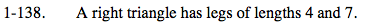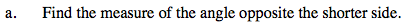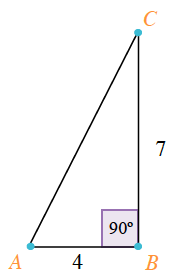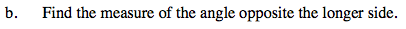### Home > PC > Chapter 1 > Lesson 1.4.1 > Problem1-138

1-138.
1. A right triangle has legs of lengths 4 and 7. Homework Help ✎

1. Find the measure of the angle opposite the shorter side.

2. Find the measure of the angle opposite the longer side.Make a sketch. What is the angle opposite the shorter side? What trig function involves this angle and the opposite and ajacent sides of it?$\text{tan }C = \frac{4}{7}\ \ \ \ \ \angle C \approx 29.74\degree$Follow a similar process as (a) above.